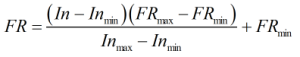# C143 – Flow Totalisation – Analogue Input

## Description

This carries out the flow rate and totals given an analogue input.

Kelton calculation reference C143

KIMS calculation reference K128

## Options

### Analogue Input

• Current
• Voltage

This option is chosen to indicate whether the input is a current or a voltage.

### Flow Rate

• Mass
• Volume
• Standard Volume

This option is used to select the type of flow rate required for output.

### Measure Input as Current/Voltage

• No
• Yes

This option allows a current/voltage reading to be input with a resistance, the range will use voltage/current(s).

### Enter elapsed time as

• Duration
• Start and end time

This option gives a choice of entering time as a duration or as the start and end time in date time format.

## Calculation

### Flow Rate

The flow rate is calculated by:Where In = Analogue input (current or voltage input) Inmin = Lower range of analogue input Inmax = Upper range of analogue input FRmin = Lower range of flow rate FRmax = Upper range of flow rate

### Totals

The totals are calculated as in C203 – Totalisation conversion using the output of the above equation and the elapsed time.

Back